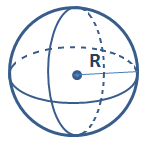Sphere Volume Calculator | Math Calculator

# Calculate Sphere Volume

Calculate Sphere Volume(Ball Volume)*

Sphere Volume Calculator